Function Repository Resource:

# RNAFoldingMaximumBasePairing

Fold a single RNA strand for maximum base pairing

Contributed by: Björn Zimmermann
 ResourceFunction["RNAFoldingMaximumBasePairing"][biosequence] determines the maximum base pairing for the RNA BioSequence strand biosequence. ResourceFunction["RNAFoldingMaximumBasePairing"][biosequence,format] gives results in the format specified by format.

## Details and Options

Base pairs are a fundamental part of RNA structures. They are formed by energetically favorable interaction known as hydrogen bonds. The more base pairs a structure contains, the more hydrogen bonds are formed. A first attempt to predict the structure of an RNA sequence could thus be to find a structure having a maximum number of base pairs. This is also known as the Nussinov algorithm.
Both Watson-Crick base pairs and GU base pairs (so-called "wobble base pairs") are supported.
Maximising the number of base pairs is too simplistic for structure prediction. Base pair stacks as a structural element provide a stabilizing effect to the structure in the free energy approach.
ResourceFunction["RNAFoldingMaximumBasePairing"] supports the following output format:
 “Compact" or Automatic (default) return the result in compact form "Expanded" return the result in expanded (Bond usage ready) form "Count" count of constructs according to Method under maximum base pairing
ResourceFunction["RNAFoldingMaximumBasePairing"] supports a Method option, which can be set to any of the following:
 "MaximumBasePairing" determine bond indices under maximum base pairing {"MaximumBasePairing",n} determine bond of n bonds out of the maximum base pairing bond list "MaximumBasePairingStack" determine bond indices of stacks under maximum base pairing {"MaximumBasePairingStack",n} determine indices of n two base pair bond stacks out of maximum base pairing bond list
The default setting is equivalent to "MaximumBasePairing".
For larger sequences potentially many lists of base pairs of the same maximum length are possible. Therefore the "Compact" output is the default: A base pair is a two argument list of bases (depth 2). Since base pairs as well as two base pair stacks are supported, single base pair 'stacks' must have the same depth as two base pair stacks (depth 3).
It is possible that in the respective part of the sequence multiple stacks are possible. These alternatives are arranged in a list (depth 4). There are such alternatives for the chosen partition of the sequence (depth 5). And finally, there could be alternative possibilities to partition (depth 6).
The "Expanded" output unravels the "Compact" format into complete lists of base pair lists, ready to use in BioSequence. Potentially this can create a very large output.

## Examples

### Basic Examples

Determine the maximum base pairing for a short RNA strand:

 In:=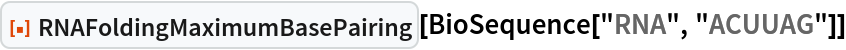Out=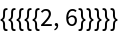Get the expanded form:

 In:=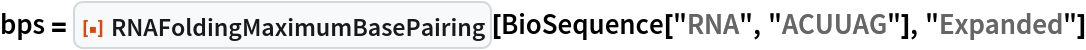Out=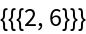Visualize the result:

 In:=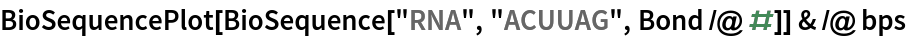Out=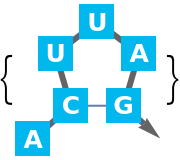Determine the number of base pairs under maximum base pairing:

 In:=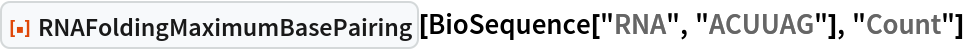Out=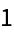Alternatives are grouped together:

 In:=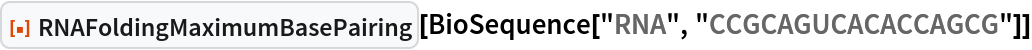Out=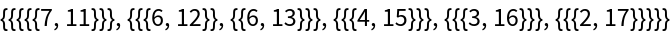Get the expanded form:

 In:=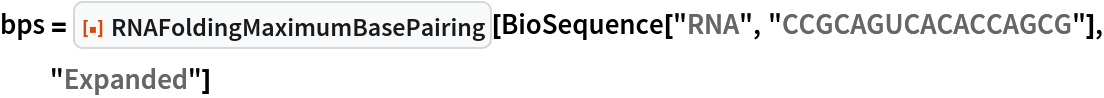Out=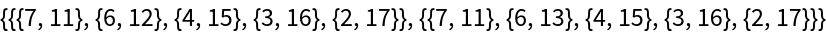Compare results side-by-side:

 In:=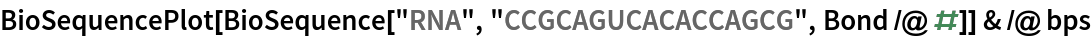Out=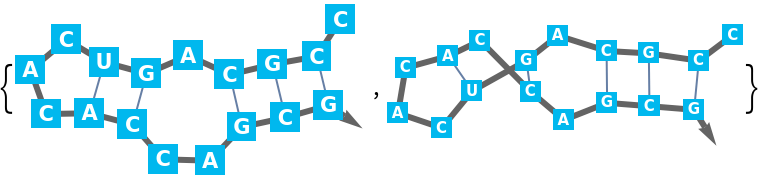Determine the number of base pairs under maximum base pairing:

 In:=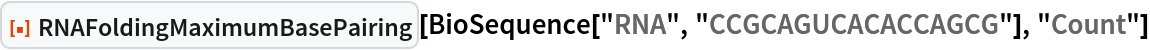Out=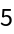Just one BioSequence of the last result contains stacks of base pairs only. These kinds can be calculated directly:

 In:=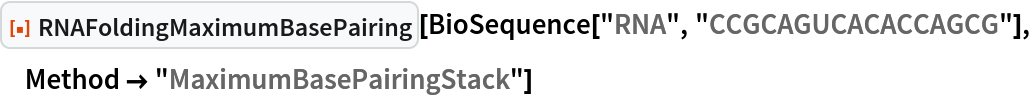Out=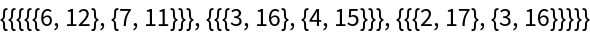Get the expanded form:

 In:=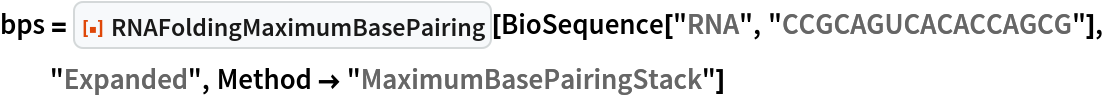Out=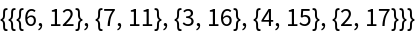Visualize the result:

 In:=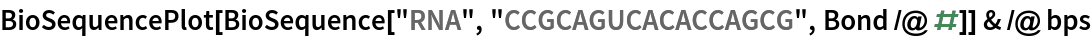Out=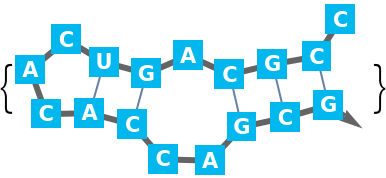Determine the number of potentially partially overlapping, two base pair stacks under maximum base pairing:

 In:=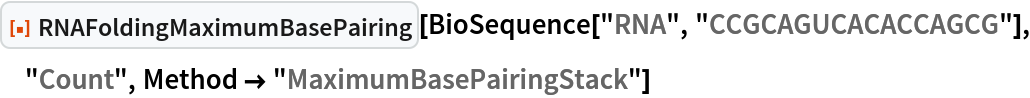Out=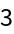## Version History

• 1.0.0 – 03 May 2022